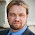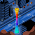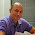Skip to main content

### A Bayes' Solution to Monty Hall

For any problem involving conditional probabilities one of your greatest allies is Bayes' Theorem. Bayes' Theorem says that for two events A and B, the probability of A given B is related to the probability of B given A in a specific way.

Standard notation:

probability of A given B is written $$\Pr(A \mid B)$$
probability of B is written $$\Pr(B)$$

Bayes' Theorem:

Using the notation above, Bayes' Theorem can be written: $\Pr(A \mid B) = \frac{\Pr(B \mid A)\times \Pr(A)}{\Pr(B)}$Let's apply Bayes' Theorem to the Monty Hall problem. If you recall, we're told that behind three doors there are two goats and one car, all randomly placed. We initially choose a door, and then Monty, who knows what's behind the doors, always shows us a goat behind one of the remaining doors. He can always do this as there are two goats; if we chose the car initially, Monty picks one of the two doors with a goat behind it at random.

Assume we pick Door 1 and then Monty shows us a goat behind Door 2. Now let A be the event that the car is behind Door 1 and B be the event that Monty shows us a goat behind Door 2. Then
\begin{aligned}
\Pr (A \mid B) &= \frac{\Pr(B \mid A)\times \Pr(A)}{\Pr(B)} \\
&= \frac{1/2\times 1/3}{1/3\times 1/2+1/3\times 0+1/3\times 1} \\
&= 1/3.
\end{aligned}The tricky calculation is $$\Pr(B)$$. Remember, we are assuming we initially chose Door 1. It follows that if the car is behind Door 1, Monty will show us a goat behind Door 2 half the time. If the car is behind Door 2, Monty never shows us a goat behind Door 2. Finally, if the car is behind Door 3, Monty shows us a goat behind Door 2 every time. Thus, $\Pr(B) = 1/3\times 1/2+1/3\times 0+1/3\times 1 = 1/2.$The car is either behind Door 1 or Door 3, and since the probability that it's behind Door 1 is 1/3 and the sum of the two probabilities must equal 1, the probability the car is behind Door 3 is $$1-1/3 = 2/3$$. You could also apply Bayes' Theorem directly, but this is simpler.

So Bayes says we should switch, as our probability of winning the car jumps from 1/3 to 2/3.

### Comments

1.This comment has been removed by the author.

2.Doesn't Pr(B) = 1/2?

[Pr(B) = 1/3 x 1/2 + 1/3 x 0 + 1/3 x 1 = 1/2]

3.Hi John! You're correct, of course. I'll correct these calculations now.

4.How to you get Pr(BIA) = 1/2?

Thx

1.This comment has been removed by the author.

5.@Mare
Pr(B|A) is the probability Monty opens Door2 given the car is behind Door1 (the door you picked). Since Monty has a choice of 2 goat doors to open in this scenario, the probability he opens Door2 is 1/2.

6.Given your interest in the quarks, may I ask if you have a view on Bayesian interpretations of quantum theory, such as QBism (http://en.wikipedia.org/wiki/QBism)? As an interested non-mathematician, I'd love to read an explanation of how QBIsm resolves "spooky" quantum theory features such as action-at-a-distance and many-state-reality, especially in the context of developments like quantum key distribution or quantum computing, which appear to use those exactly those features.

1.Statistical/Math Bayes has nothing in common with QBism except for the appellation.

7.This comment has been removed by the author.

8.This comment has been removed by the author.

9.Your error is here: "and since the probability that it's behind Door 1 is 1/3 ".

This is not true. The probability that it's behind Door 1 changes to 1/2 when a third of the probabilities are eliminated by opening Door 2.

Talk about mass hypnosis.

1."The probability that it's behind Door 1 changes to 1/2 ..."
I would be interested to see a Bayesian explanation for that.

2.This comment has been removed by the author.

3.This comment has been removed by the author.

10.This comment has been removed by the author.

11.This comment has been removed by the author.

12.Hi Christopher,

If we define C as Contestant selects Door #1

Isn't the value you calculated as Pr(B) in actuality Pr(B|C)? Shouldn't we use Pr(B) without condition C?

Regards,
Tom

13.This is a rather strange and unnecessary use of Bayes' theorem.
You really didn't need Bayes to conclude that the probability of the car being behind the door you (initially) picked is 1/3. This is given! And in fact you use this same probability as "P(A)".

The fact that Monty does "B" doesn't matter to event "A" (there's either a car behind door 1 or not, no matter what Monty does afterwards (!) with the remaining doors), so "P(A|B)" and "P(A)" must always be equal here.

So your explanation of the Monty Hall problem really just starts being interesting/correct and addressing the problem at
"The car is either behind Door 1 or Door 3, and since ..."

14.quite an easy puzzle

15.quite an easy puzzle

### Mixed Models in R - Bigger, Faster, Stronger

When you start doing more advanced sports analytics you'll eventually starting working with what are known as hierarchical, nested or mixed effects models. These are models that contain both fixed and random effects. There are multiple ways of defining fixed vs random random effects, but one way I find particularly useful is that random effects are being "predicted" rather than "estimated", and this in turn involves some "shrinkage" towards the mean.

Here's some R code for NCAA ice hockey power rankings using a nested Poisson model (which can be found in my hockey GitHub repository):
model <- gs ~ year+field+d_div+o_div+game_length+(1|offense)+(1|defense)+(1|game_id) fit <- glmer(model, data=g, verbose=TRUE, family=poisson(link=log) ) The fixed effects are year, field (home/away/neutral), d_div (NCAA division of the defense), o_div (NCAA division of the offense) and game_length (number of overtime periods); off…

### Notes on Setting up a Titan V under Ubuntu 17.04

I recently purchased a Titan V GPU to use for machine and deep learning, and in the process of installing the latest Nvidia driver's hosed my Ubuntu 16.04 install. I was overdue for a fresh install of Linux, anyway, so I decided to upgrade some of my drives at the same time. Here are some of my notes for the process I went through to get the Titan V working perfectly with TensorFlow 1.5 under Ubuntu 17.04.

Old install:
Ubuntu 16.04
EVGA GeForce GTX Titan SuperClocked 6GB
2TB Seagate NAS HDD
+ additional drives

New install:
Ubuntu 17.04
Titan V 12GB
/ partition on a 250GB Samsung 840 Pro SSD (had an extra around)
/home partition on a new 1TB Crucial MX500 SSD
New WD Blue 4TB HDD
+ additional drives

You'll need to install Linux in legacy mode, not UEFI, in order to use Nvidia's proprietary drivers for the Titan V. Note that Linux will cheerfully boot in UEFI mode, but will not load any proprietary drivers (including Nvidia's). You'll need proprietary drivers for Tens…